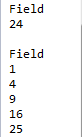# 游标归并与连接

## 有序归并

 A B C 1 =file("Order_Wines.txt") 2 =file("Order_Electronics.txt") 3 =file("Order_Foods.txt") 4 =file("Order_Books.txt") 5 =[A1:A4].(~.cursor@t()) 6 =A5.conjx() 300 7 for =A6.fetch(B6) 8 if B7==null break 9 =B7(1).Type =B7(B6).Type 10 if B9!=C9 break 11 >A6.close()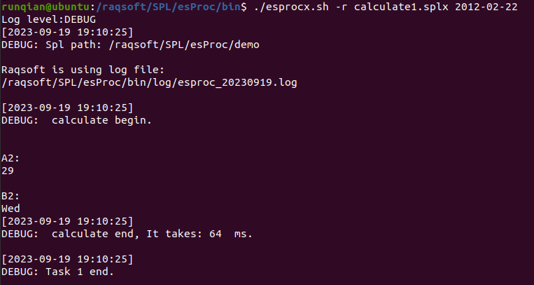A B 1 =file("Order_Wines.txt") 2 =file("Order_Electronics.txt") 3 =file("Order_Foods.txt") 4 =file("Order_Books.txt") 5 =[A1:A4].(~.cursor@t()) 6 =A5.mergex(Date) 300 7 for =A6.fetch(B6) 8 break 9 >A6.close()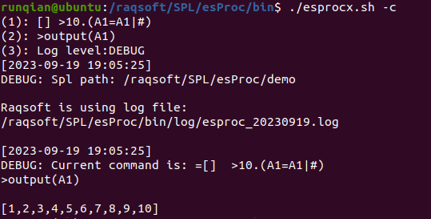A B 1 =file("Order_Wines.txt") 2 =file("Order_Electronics.txt") 3 =file("Order_Foods.txt") 4 =file("Order_Books.txt") 5 =[A1:A4].(~.cursor@t().sortx(PID)) 6 =A5.mergex(PID) 2000 7 for =A6.fetch(B6) 8 break 9 >A6.close()

B7中，读取出的记录如下：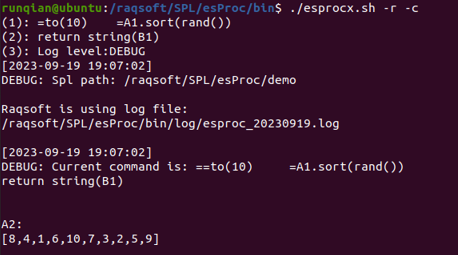## 对齐式连接

 A 1 =file("Order_Wines.txt").cursor@t() 2 =file("Order_Electronics.txt").cursor@t() 3 =file("Order_Foods.txt").cursor@t() 4 =file("Order_Books.txt").cursor@t() 5 =A1.groupx(Type,Date;count(~):Count,sum(round(decimal(Amount),2)):TotalAmount;100) 6 =A2.groupx(Type,Date;count(~):Count,sum(round(decimal(Amount),2)):TotalAmount;100) 7 =A3.groupx(Type,Date;count(~):Count,sum(round(decimal(Amount),2)):TotalAmount;100) 8 =A4.groupx(Type,Date;count(~):Count,sum(round(decimal(Amount),2)):TotalAmount;100) 9 =joinx@1(A5:Wines,Date;A6:Electronics,Date;A7:Foods,Date;A8:Books,Date) 10 =A9.fetch(25) 11 >A9.close()

A5~A8中，分别对每一类产品分类聚合运算，各自返回缓存文件游标。关于groupx的使用，在中有更详细的说明。在A9中将各类产品每日的销售数据按照日期对齐连接。在A10中取出前25天的统计结果如下：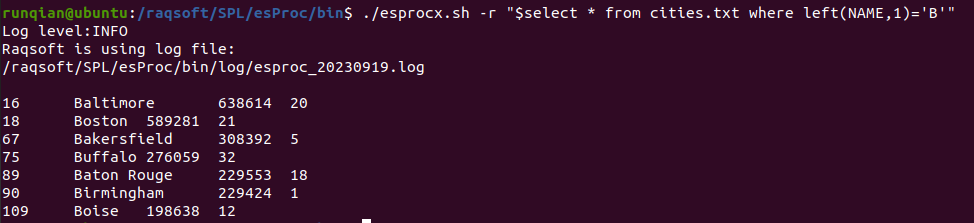9 =joinx@1(A5:Wines,Date;A6:Electronics,Date;A7:Foods,Date;A8:Books,Date) 10 =A9.select(Foods.TotalAmount>Wines.TotalAmount) 11 =A10.new(Foods.Date:Date,Foods.TotalAmount:Foods,Wines.TotalAmount:Wines) 12 =A11.fetch(100) 13 >A11.close()

A10在连接的游标中，添加计算筛选出食品订单总额大于酒类订单总额的记录，并在A11中继续添加计算生成结果。在A12中返回了前100条结果，如下：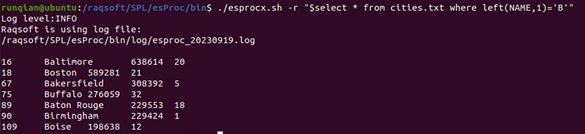A 1 \$select * from CITIES 2 \$select * from STATES 3 =A1.cursor() 4 =A2.cursor() 5 =joinx(A3:City,STATEID;A4:State,STATEID) 6 =A5.fetch()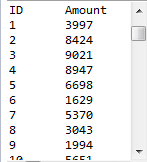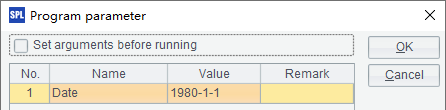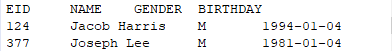A 1 \$select * from CITIES order by STATEID 2 \$select * from STATES order by STATEID 3 =A1.cursor() 4 =A2.cursor() 5 =joinx(A3:City,STATEID;A4:State,STATEID) 6 =A5.fetch()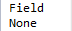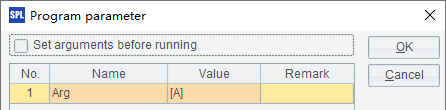A 1 =file("PersonnelInfo.txt") 2 =A1.cursor@t().sortx(State) 3 \$(demo) select STATEID, NAME, ABBR from STATES order by ABBR 4 =A3.cursor() 5 =joinx(A2:PersonnelInfo,State;A4:State,ABBR) 6 =A5.fetch(100) 7 >A5.close()

A5中将员工信息与对应的州记录连接。需要注意的是，连接之前要保证游标中的数据完成了排序。A6中获取前100条数据如下：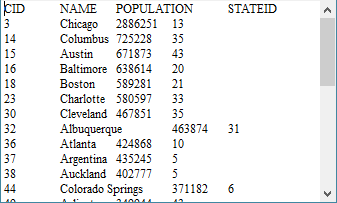A 1 =file("PersonnelInfo.txt") 2 =A1.cursor@t(ID,Name,City,State) 3 \$(demo) select * from STATES order by ABBR 4 =file("StateFile.btx") 5 >A4.export@z(A3;ABBR) 6 =A2.joinx(State,A4:ABBR,NAME:SName,CAPITAL:SCapital;10000) 7 =A6.fetch(100) 8 >A6.close()

A5中用数据库中的州信息生成可分段集文件StateFile.btx，注意集文件需要按照连接时所需使用的键来排序，在这个例子中集文件对ABBR字段是有序的。在A6中，用函数cs.joinx(C:…, f:K:…, x:F,…;…;n)来将游标与集文件连接。连接时，用游标cs的字段C:…与集文件f的键K:匹配，从而从集文件中找到对应的记录，并用记录计算出表达式x的结果作为字段F添加到游标中。最后的参数是缓冲区行数，用来设定计算中使用缓存文件的最大行数。使用cs.joinx()时只需要求集文件有序，对游标中的数据没有顺序要求。具体到上面的例子中，A6中的函数用来根据员工所在州的简称，从州数据集文件中找到对应的州记录，并从中取出州的全称和首都，在游标中生成两个新的字段SNameSCapitalA7中获取前100条数据如下：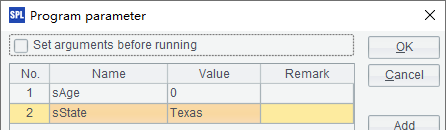A 1 =file("PersonnelInfo.txt") 2 =A1.cursor@t() 3 \$(demo) select STATEID, NAME,ABBR from STATES 4 =create(Month,State,GroupID) 5 >A3.(12.((m=~,A4.insert(0,m,A3.ABBR,A3.ABBR+string(m))))) 6 =A2.join(month(Birthday):State,A4:Month:State,GroupID:Group) 7 =A6.fetch(100) 8 >A6.close()

A4中根据月份及州的简称创建序表，A5填入数据后，A4中序表如下：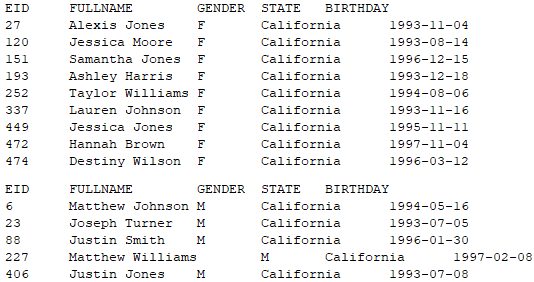A6中，通过连接的方式，在游标A2中添加字段，添加时，用A2游标中的员工生日月份及State，和序表A4中的MonthState做等值连接，连接后，取出A4x序表中的GroupID字段添加到结果中的State字段中。从A7中读取前100条结果如下：